International Journal of Mineral Processing and Extractive Metallurgy
Volume 1, Issue 1, March 2016, Pages: 1-7

The Management of Lead Concentrate Acquisition in "Trepca"

Ahmet Haxhiaj1, Maoming Fan2, Bajram Haxhiaj3

1Faculty of Geosciences, University of Mitrovca "Isa Boletini", Mitrovica, Republic of Kosovo

2Research and Development Department, Eriez Manufacturing Co. Erie, Pennsylvania, USA

3Faculty of Civil Engineering and Architecture, University of Prishtina "Hasan Prishtina", Prishtina, Republic of Kosovo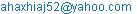(A. Haxhiaj)

Ahmet Haxhiaj, Maoming Fan, Bajram Haxhiaj. The Management of Lead Concentrate Acquisition in "Trepca". International Journal of Mineral Processing and Extractive Metallurgy. Vol. 1, No. 1, 2016, pp. 1-7. doi: 10.11648/j.ijmpem.20160101.11

Abstract: Based on the placement of lead and its consumption in industry branches, the paper deals with the composition of lead in the massive of Kopaonik. Removal, tenuity and grinding of galenit. The paper in particular treats the flotation process. Also treats physico-chemical bases of reagents in the process, as well as decanting, drying, filtering and storage of concentrates. Verification of the chemical composition of Pb concentrates with Pb, Zn, Bi, As, Sb, In, Au, Ag, etc. It is special that in Kopaonik massive the ratio of Pb: Zn composition is 1.4: 1.0 and during the flotation lead is inclined to go with pirotines concentrate more than is allowed. We are strongly focused to maximally decrease the Pb amount that goes with pirotine concentrates and wastes. The paper as such gave the first effects in optimizing of these parameters with positive effects in flotation process in Trepca.

Keywords: Mineral, Reagents, Grinding, Flotation, Pb Concentrate, Pirotine, Sterile

1. Introduction

2. From Literature

2.1. Froth Flotation – Fundamental Principles

Froth flotation is a highly versatile method for physically separating particles based on differences in the ability of air bubbles to selectively adhere to specific mineral surfaces in a mineral/water slurry. The particles with attached air bubbles are then carried to the surface and removed, while the particles that remain completely wetted stay in the liquid phase. Froth flotation can be adapted to a broad range of mineral separations, as it is possible to use chemical treatments to selectively alter mineral surfaces so that they have the necessary properties for the separation. It is currently in use for many diverse applications, with a few examples being: separating sulfide minerals from silica gangue (and from other sulfide minerals); separating potassium chloride (sylvite) from sodium chloride (halite); separating coal from ash-forming minerals; removing silicate minerals from iron ores; separating phosphate minerals from silicates; and even non-mineral applications such as de-inking recycled newsprint. It is particularly useful for processing fine-grained ores that are not amenable to conventional gravity concentration.

2.2. Particle/Bubble Contact

Once the particles are rendered hydrophobic, they must be brought in contact with gas bubbles so that the bubbles can attach to the surface. If the bubbles and surfaces never come in contact, then no lotation can occur. Contact between particles and bubbles can be accomplished in a flotation cell such as the one shown schematically in Figure 1.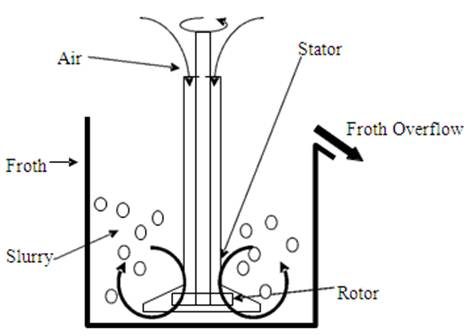Figure 1. Simplified schematic of a conventional flotation cell. The rotor draws slurry through the stator and expels it to the sides, creating a suction that draws air down the shaft of the stator. The air is then dispersed as bubbles through the slurry, and comes in contact with particles in the slurry that is drawn through the stator. Particle/bubble collision is affected by the relative sizes of the particles. If the bubbles are large relative to the particles, then fluid flowing around the bubbles can sweep the particles past without coming in contact. It is therefore best if the bubble diameter is comparable to the particle diameter in order to ensure good particle/bubble contact.

2.3. Conventional Cells

Conventional flotation cells consist of a tank with an agitator designed to disperse air into the slurry, as was previously shown schematically in Figure 5. These are relatively simple machines, with ample pportunity for particles to be carried into the froth along with the water making up the bubble films (entrainment), or for hydrophobic particles to break free from the froth and be removed along with the hydrophilic particles. It is therefore common for conventional flotation cells to be assembled in a multi-stage circuit, with "rougher", "cleaner", and "scavenger" cells, which can be arranged in configurations such as the one shown in Figure 2.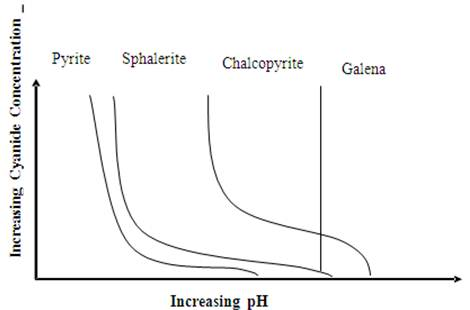Figure 2. One possible configuration for a Rougher/Cleaner/Scavenger flotation circuit.

2.4. Reagents

The properties of raw mineral mixtures suspended in plain water are rarely suitable for froth flotation. Chemicals are needed both to control the relative hydrophobicities of the particles, and to maintain the proper froth characteristics. There are therefore many different reagents involved in the froth flotation process, with the selection of reagents depending on the specific mineral mixtures being treated.

The most common collectors for sulfide minerals are the sulfhydryl collectors, such as the various xanthates and dithiophosphates. Xanthates are most commonly used, and have structures similar to what is shown in Figure 3. Xanthates are highly selective collectors for sulfide minerals, as they chemically react with the sulfide surfaces and do not have any affinity for the common non-sulfide gangue minerals. Other highly-selective collectors for use with sulfide minerals, such as ithiophosphates, have somewhat different adsorption behavior and so can be used for some separations that are difficult using xanthates.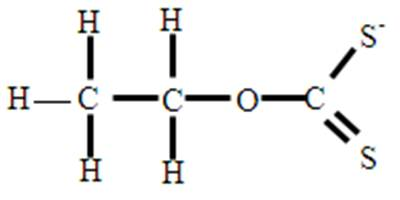Figure 3. Structure of a typical xanthate collector (ethyl xanthate). The OCSS- group attaches irreversibly to the sulfide mineral surface. Using xanthates with longer hydrocarbon chains tends to increase the degree of hydrophobicity when they adsorb onto the surface.

3. The Management of Lead Mineral Resources

Place resources of Pb ores are composite place resouces, that exept Pb minerals also contain significant quantity of Zn minerals. So in most cases when it comes to Pb ores we mean lead and zinc ores. In smaller quantity are also present other metal minerals as Au and Ag minerals, Fe minerals, Cu minerals, Bi minerals, etc.

Table 1. Presents the chemical composition of lead ores in Kopaunik massif.

 Type of mineral % Pb %Zn g/ tAg %Fe %Cu %S % SiO2 1 5.5 13.0 - 9.4 - - 18.0 2 8.5 13.8 - 1.8 1.0 - 20.0 3 6.0 13.1 - - - 16.0 19.0 4 4.0 2.8 60 - - - - 5 2.8 2.1 51 - - - - 6 3.7 2.4 52 - - - -

Trepca's mine has modern infrastructure based on contemporary underground mining model. It has three wells developed in 12 horizons, while in the last three horizons are developed ramps that enable application of modern mechanisms for preparing the ore.

Mine with its infrastrukture as well as with modern methods of filling, has the capacity of ore use from 500000 up to 650000 tons a year.

3.1. Galenit Quality

Lead with sulfur forms only a component which can be found in natyre also in the form of clear mineral, as dark colored and metal shining Galenit. Temperature of its melting is 1135°C. In absence of air the galena starts to sublimate in temperature about 7500°C. Galena belongs to the group of components that evaporate easily and this attribute is the major cause of lead losses during its processing. Place resources of lead in Kosovo lie in the so-called metallurgical belt of Trepca, which is situated in the northeast of Kosovo starting from Leposavic to Gjilan. Length of the belt is over 80km and average width of it is 30km.

Table 2. Presents total ore reserves and the quantity of lead and ore in metallurgical belt of Trepca.

 Resource place Ores(ton) % Pb Pb metal in(ton) Stan Terg 35081000 3.85 1349579 Cerrnac BBGom 7544227 6.85 516645 ArtanesCB complex 16837227 4.67 749354 Total 58662569 4.46 2615578

3.2. Flotation Process

The ore from Stan Terg mine is carried by train to flotation, which has enough capacity for Stan Terg mine. Flotation process includes three important stages that are:

1.   Grinding and teunity,

2.   Classification of sizes, and

3.   Conditioning.

Finalized grinding and teunity we consider it when the mineral derived from mine with size 400mm is teunited in the size 0.074µm. The broken mineral in 0.6mm together with reagents that weight 74t/h is dosed in mill for final teunity. Measure/Pulp in particles with diameter 0.074µm from mill with funnel Φ= 100 mm is carried to lead conditioner. Flotation process of minerals is based on Physical-chemical attributes of each mineral phase and their interaction laws. The basic goal of the flotation proces is selective seperation of useful compounds from the others. To achieve such a goal it is necessary that the mineral components of one ore, to remain in abeyance in aquatic environment. While being at the same time smooth/distributed together with air bubbles sent to this environment. Under conditions of such a mix where is puted into motion the phases dynamic, stiff, liquid, flotation environment gases, also happens selective seperation of minerals where a part of them is attached to bubbles and the other part remains in water.

Bubbels/Aggregate (Froth) - Bubbels and mineral grindings have a smaller density than aqueous environment and get in surface of pulp by forming the product of flotation process(concentrate). The mineral particles interact with air bubbles, is possible in those cases where surface virtues of these particles have hydrophobic and natyral abilities, or created by the activity and impact of reagents used in flotation process.

Table 3. Presents the type and quantity of reagents used in flotation process of lead mineral.

 Reagents Concentration kg t-1 Foams 0.012-0.12 Collectors 0.02-1.2 Carbohydrate oil 0.5-2.5 pH regulators 0.55-5.0 Activators 0.025-1.0 Depressors 0.025-0.5 Deactivators 0.025-0.25

In general reagents are seperated in three groups: collecting reagents, fixing reagents, and foam making reagents. Their use is very important in idustry.

Flotation process of lead mineral has three main processes: base flotation, controlling flotation and cleaning flotation.

Base and controlling flotation is done in pneumatic machines with "Denver" imperller type. In series are established 12 cells, each cell has a volume V=2.83m3. Scavenger flotation is done in mechanical machines with impeller and air. The machine has 14 cells, each cell has a volume V=1.1m3. The whole technological process of flotation is presented in .

Teunity mass 0.074 µm as digestion is dosed in base flotation in the machine with 12 cells, and surface product with the help of shovels is carried to machine number 8 of digestion flotation. While the lower leakage part is carried to entrance of controlling flotation for reflotation. The controlling flotation product is managed as follows. Quantity of first 6 cells is carried in cell number 4 of digestion flotation as final product (concentrate), while the product of 6 other cells of controlling flotation is returned in conditioner. Whereas the low mass of 6 cells of controlling flotation, goes as sterile.

4. Experimental Part

4.1. Mathematical Models for Calculating the Flotation Product

There is no one universal method for expressing the effectiveness of a separation, but there are several methods that are useful for examining froth flotation processes:

(a).     Ratio of Concentration, the weight of the feed relative to the weight of the concentrate, The Ratio of Concentration is F/C, where F is the total weight of the feed and C is the total weight of oncentrate. While this data is available in laboratory experiments, in the plant it is likely that the ore is not weighed and only assays will be available. However, it is possible to express the ratio of concentration in terms of ore assays. Starting with the mass balance equations, and the definition of the ratio of concentration:

F = C + T, Ff = Cc + Tt, Ratio of Concentration = F/C

where F, C, and T are the % weights of the feed, concentrate, and tailings, respectively; and f, c, and t are the assays of the feed, concentrate, and tailings. We now need to eliminate T from these equations so that we can solve for F/C:

Ff = Cc + Tt, and multiplying (F = C + T) by t gives us:

Ft = Ct + Tt, so subtracting this equation from the previous eliminates T and gives:

F(f - t) = C(c - t), and rearranging produces the equation for the ratio of concentration:

F/C = (c – t)/(f – t)                            (1)

(b).     % Metal Recovery, or percentage of the metal in the original feed that is recovered in the concentrate. This can be calculated using weights and assays, as (Cc)/(Ff)·100. Or, since C/F = (f – t)/(c – t), the % Metal Recovery can be calculated from assays alone using 100(c/f)(f – t)/(c –t).

(c).      % Metal Loss is the opposite of the % Metal Recovery, and represents the material lost to the tailings. It can be calculated simply by subtracting the % Metal Recovery from 100%.

(d).     % Weight Recovery is essentially the inverse of the ratio of concentration, and equals

100·C/F = 100·(f – t)/(c – t).

(e).      Enrichment Ratio is calculated directly from assays as c/f, weights are not involved in the calculation.

The main parameters of technological process of lead flotation in "Trepca" company

F = 150 t h-1 load dosed for flotation.

C- concentrate weight that depends on quality of technological flotation process.

T- losess weight, sterility that depends on the quality of mineral and in technological flotation process.

f- percentage of steel in ore.

c- percentage of steel in concentrate that depends on the quality of technological flotation process.

t- percentage of steel in sterile that depends on the quality of technological flotation process.

Alternative I, the calculation of lead concentrate quantity, Pb and Zn's percentage in concentrate and Pb losess with residu when mineral contains 3.17%Pb.

a    The quantity of Pb concentrate is calculated with mathematical formula.

F/C = (c – t)/(f – t)                             (1)

F = 150 t

Laboratory analysis of mineral, concentrate and sterile made in the Laboratory of Trepca's company dated 18.06.2013, results:

f = 3.17%Pb

c = 75.3 % Pb

t = 0.29 % Pb

C= F(f-t) / (c-t) = 150 (3.17- 0.29) / (75.3-0.29) = 5.7993 t h-1

C = 5.7993 t h-1

b    The quantity of Pb in Pb concentrate is calculated with mathematical formula.

CPb = C x c t.h-1                               (2)

CPb = 5.7993 x 0.753 t.h-1

CPb = 4.366 t h-1

c     Pb quantity in losess that goes with Zn concentrate with pirot and sterile, is calculated with mathematical formula.

Closess = CPb mineral—CPb t h-1                  (3)

Closess =4.755-4.366 t h-1

Closess = 0.389 t h-1

d    % Weight Recovery is essentially the inverse of the ratio of concentration, and equals mathematical formula.

100·C/F = 100·(f – t)/(c – t).                    (4)

100·C/F - 100·(f – t)/(c – t)= C/F - (f – t)/(c – t) = 0.00023%

0.00023 % is Pb concentration from load.

e     Mineral enrichment, load is calculated with mathematical formula.

c/f %                                         (5)

75.3/3.17= 23.753% is solid enrichment of mineral

f     Losess weight is calculated with mathematical formula.

F = C + T t h-1                                 (6)

T= F - C t h-1

T = 150 - 5.7993 = 144.200 t h-1

144.200 t h-1 is losess weight which in it self contains Zn concentrate, pirot concentrate and the sterile.

g     The lead enrichment report is calculated with mathematical formula.

REnrichment Pb = %Pbkoncentrat / %Pbmineral                   (7)

REnrichment Pb = 75.3/ 3.17 = 23.753 %

REnrichment Pb = 23.752 % is lead enrichment during flotation process

Alternative II, the calculation of lead concentrate quantity, percentage of Pb in Pb and Zn’s concentrate and Pb losess with residu when mineral contains 3.17 % Pb.

a    The quantity of Pb concentrate is calculated with mathematical formula (1).

F = 150 t

Concentrate laboratory analysis of mineral, and sterile made in the" Laboratory of Trepca"'s company dated 19.06.2013, results:

f= 3.17%Pb

c = 73.60 % Pb

t = 0.14 % Pb

C= F (f-t)/(c-t) = 150 (3.17-0.14)/(73.60-0.14) = 6.207 t h-1

C = 6.207 t h-1

b    The quantity of Pb in Pb concentrate is calculated with mathematical formula (2).

CPb = 6.207 x 0.736 t.h-1

CPb = 4.568 t h-1

c     Pb quantity in losess that goes with Zn concentrate with pirot and sterile, is calculated with mathematical formula (3).

Cmbetje=4.755-4.568 t h-1

Cmbetje= 0.167 t h-1

d    % Weight Recovery is essentially the inverse of the ratio of concentration, and equals mathematical formula (4).

100·C/F - 100·(f – t)/(c – t)= C/F - (f – t)/(c – t) = 6.207/ 150 - (3.17 – 0.14) / (73.60 – 0.17) = 0.00018 %

0.00 018 % Pb concentration from load.

e     Mineral enrichment, load is calculated with mathematical formula (5).

73.60/3.17= 23.217 % solid enrichment of mineral

f     Losess weight is calculated with mathematical formula (6).

T= F - C t h-1

T = 150 – 6.207 = 143.793 t h-1

143.793 t h-1 is losess weight which in it self contains Zn concentrate, pirot concentrate and the sterile.

g     The lead enrichment report is calculated with mathematical formula (7).

REnrichment Pb = 73.60 / 3.17 = 23.217 %

REnrichment Pb = 23.217 % is lead enrichment during flotation process

Alternative III, the calculation of lead concentrate quantity, percentage of Pb in Pb and Zn’s concentrate and Pb losess with residu when mineral contains 4.67 % Pb and it has been taken from "Artanes" ore.

a    Quantity of Pb concentrate is calculated with mathematical formula (1).

F = 150 t

Laboratory analysis of mineral, concentrate and sterile made in the Laboratory of Trepca's company dated 20.06.2013, results:

f= 4.67%Pb

c = 74.4 % Pb

t = 0.29 % Pb

C= F(f-t)/ (c-t) = 150 (4.67- 0.29) / (74.4-0.29) =8.865 t h-1

C = 8.865 t h-1

b    The quantity of Pb in Pb concentrate is calculated with mathematical formula (2).

CPb = 8.865 x 0.744 t.h-1

CPb = 6.595 t h-1

c     Pb quantity in losess that goes with Zn concentrate with pirot and sterile, is calculated with mathematical formula (3).

Closess=7.005 - 6.595 t h-1

Closess= 0.47 t h-1

d    % Weight Recovery is essentially the inverse of the ratio of concentration, and equals mathematical formula (4).

100·C/F - 100·(f – t)/(c – t)= C/F - (f – t)/(c – t) = 8.865 / 150 - (4.67 – 0.29) / (74.4 – 0.29) = 0.0060 %

0.0060 % Pb enrichment from load.

e     Mineral enrichment, load is calculated with mathematical formula (5).

74.4/4.67 = 15.931 % is a not good enrichment of mineral

f     Losess weight is calculated with mathematical formula (6).

T= F - C t h-1

T = 150 – 8.865 = 141.135 t h-1

141.139 t h-1 is losess weight which in it self contains Zn concentrate, pirot concentrate and the sterile.

g     The lead enrichment report is calculated with mathematical formula (7).

REnrishment Pb = 74.4 / 4.67 = 15.931 %

REnrishment Pb = 15.931 % is a not good enrichment of Pb during flotation process.

Table 4. Presents quantity of Pb in mineral, quantity of Pb concentrate, quantity of Pb in concentrate and Pb quantity in losess for Alt. I, II, III.

 Alternatives Quantity of Pb in mineral t h-1 Quantity of Pb concentrate t h-1 Quantity of Pb, in Pb concentrate t h-1 Quantity of Pb in losess t h-1 Alt.I 4.753 5.7993 4.366 0.389 Alt.II 4.753 6.207 4.568 0.167 Alt.III 7.005 8.865 6.595 0.47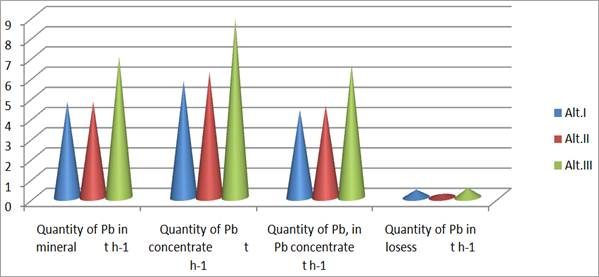Figure 4. Presents quantity of Pb in mineral, quantity of Pb concentrate, quantity of Pb in concentrate and Pb quantity in losess for Alt. I, II, III.

Table 5. Presents Pb and mineral enrichment in Alt. I II, III.

 Alternative Mineral enrichment % Pb enrichment % Alt. I 23.753 23.752 Alt. II 23.217 23.217 Alt. III 15.931 15.931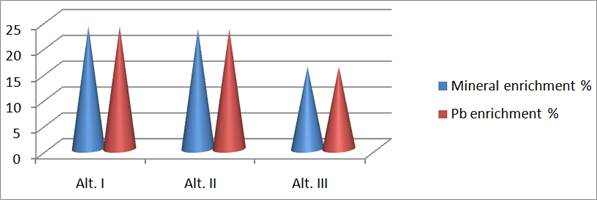Figure 5. Presents Pb and mineral enrichment in Alt. I II, III.

4.2. Ag Quantity in Lead Minerals

Sulfur minerals of Pb-Zn are rich with precious metals. Chemical analysis in the laboratory of Trepca's company dated 19.06.2014, result as below.

Table 6. Presents the composition of some samples.

 Sample Shift Quantity Ag t-1 1 1 11.38 2 2 38.50 3 3 16.67

Ag quantity in mineral is calculated with mathematical expression.

CAg = F x g t-1 Agmineral                         (8)

Ag quantity for the first sample is.

CAg = 150 t h-1 x 11.38g t-1

CAg = 0.001707 t h-1

CAg = 1.707 kg h-1

Ag quantity for the second sample is.

CAg = 150 t h-1 x 38.50 g t-1

CAg = 0.05775 t h-1

CAg = 57.750 kg h-1

Ag quantity for the third sample is.

CAg = 150 t h-1 x 16.67 g t-1

CAg = 0.0250 t h-1

CAg = 25.005 kg h-1

5. Discussion of Results

Based on idustrial work of technological flotation process in "Trepca", and laboratory analysis we notice main parameters which have functional/management power in flotation process. In Alt. I and II is treated Pb mineral with chemical composition of 3.17% Pb. And we notice that:

Quantity of Pb in mineral is 4.755 t h-1.

Quantity of Pb concentrate and quantity of Pb in Pb concentrate for Alt. II has an Increase with value C= 0.4053 t h-1, while Pb quantity in Pb concentrate han an increase with vlaue CPb = 0.202 t h-1.

Quantity of Pb losess for Alt. II has a decrease with value 0.222 t h-1

Mineral enrichment for Alt. II has a decrease with value 0.536 t h-1

Losess weight for Alt. II has a decrease with value 0.407 t h-1

Pb enrichment during the flotation process in Alt. II has a decrease with value 0.535%

In Alt. III the flotation process has used mineral of Artana which has 4.67 % Pb and based in production of the flotation process in "Trepca" and laboratory analysis we notice:

Quantity of Pb concentrate and quantity of Pb in Pb concentrate increases with average value C = 2.961 t h-1 and CPb = 2.192 t h-1.

Quantity of Pb in losess increases with average value Closese = 0.8455 t h-1

Enrichment of Pb during flotation process fol Alt. III has a decrease with average value Renrichment Pb =7.5315%. These parameters of technological flotation process identify a good management and a not good management of flotation process of Pb mineral in "Trepca".

6. Conclusions

Based in industrial process of flotation and in calculations made, can be concluded that all the balance positions of lead concentrate are variable. Losess and residu of lead in losess have a high value in technological process of flotation. To have minimum loss of lead in residu the temperature of mass in flotation, grinding, classification, conditioning, Ph and drying should be continual and as such the process is qualitative.

The values derived form the industrial process of flotation and laboratory analysis we can conclude that the process of flotation in Alt. II has a decrease of Pb quantity that goes with losess, as such Alt. II has a positive impact on economical sustainability in flotation procesess in Trepca.

In Alt. III, in flotation process we have used the mineral with composition 4.67% Pb. Quantity of mineral and main parameters of flotation process as grinding, conditioning, Ph and drying are the same as in Alt. I, II.

Based on the product of flotation process and laboratory analysis we can conclude:

Pb quantity dosed in flotation process with mineral is 7.005 t h-1 which is bigger than in Alt. I, II for 2.25 t h-1. Quantity of Pb concentrate has an increase with average value 2.851 t h-1 toward Alt. I, II. Quantity of Pb in losess as an increase with average value 0.192 t h-1, a quantity that has a bad impact on economical sustainability in flotation procesess in Trepca.

Chemical percentage of Pb in mineral must be in borders 3.17% ≤ %Pb ≤ 4.67%.

Conclusion is made by laboratory and graphic analysis, and also by losess of lead with residu with values from 0.167 t h-1 until 0.471 t h-1, wich is a crucial parameter for managerial quality of flotation proces in Trepca.

References

1. Froth Flotation – Fundamental Principles.
2. Kawatra, S. K., and Eisele, T. C., (1992) "Recovery of Pyrite in Coal Flotation: Entrainment or Hydrophobicity?" Minerals and Metallurgical Processing, Vol. 9, No. 2, pp. 57-61.
3. Kawatra, S. K., and Eisele, T. C. (2001), Coal Desulfurization: High-Efficiency Preparation Methods, Taylor and Francis, New York, 360 pages.
4. AGOLLI F. (1985) Metalurgjia e metaleve me ngjyrë, UP Prishtinë.
5. BITTRICH J. H. (1976) Leitdaten der chemischen thermodynamic, Berlin.
6. HAXHIAJ A., ELEZI D., SHKOLOLLI Sh. (2006) Mjedisi dhe menaxhimi i gazrave termike në zonën e parangrohjes të furrave Vatergjakete në Trepçë. Simpozium VI, Materials and their use, Tiranë.
7. HAXHIAJ A. (1989) Materialna i toplotna bilansa PORTPIRI pec´i, magistarski rad, Sveučilište u Zagrebu, MF u Sisku.
8. Haxhiaj A., Rizaj M., Elezi D. (2007) The management of coke and the optimum percentage of lead in agglomerate in Port-Piri furnace. 10TH National conference of Metallurgy, Bullgari.
9. HAXHIAJ, A. (2003) Intensifikimi dhe optimizimi I procesit të shkrirjes reduktuese të aglomeratit të plumbit në furrat shahte, punim i doktoratës, UP FXM, Mitrovicë.
10. JOVANOVIC, M. (1978) Nova tehnologija i pos trojenja za proizvodnja olova, Zbornik radova II Jugoslovenskog simpozijuma o metalurgij, TMF (8, 31, 51) Beograd.
11. RIZAJ M., TERZIQI A., BEQIRI E., KAMBERAJ N. (2008) Concentration and Distribution Characteristics ofTrepça Shaft Furnace Slag, World of Metallurgy-Erzmetall 61 N0. 2, 109-114, Clausthal-Zellerfeld, Germany.
12. Haxhiaj A, and Haxhiaj E, (2010) The Optimization of the Coke and Agglomerate Quantity in Lead Production in"Water-Jacket" Furnace, TMS Annual Meeting & Exhibition, Seattle Washington, 249-257.

 Contents 1. 2. 2.1. 2.2. 2.3. 2.4. 3. 3.1. 3.2. 4. 4.1. 4.2. 5. 6.
Article ToolsAbstractPDF(302K)